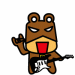2018 iT 邦幫忙鐵人賽DAY 25
2
Modern Web

[PixiJS - Day-25] 實作：基本繪圖、動態與使用 dat.GUI 測試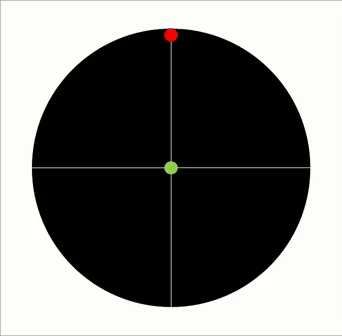Step1. 畫出線段與球：

• 畫出一顆球與線段
• 放進場景上
• 將場景放到畫面正中間

**Step2. 讓球動：**由於不需要複雜的運動，直接寫在 app.ticker 的呼叫裡就好

**Step3. 複製線段與球：**依據設定，將線段與球等分複製到不同角度

**Step4. 調整運動的參數：**不同的線段裡的球，簡諧運動的參數不太相同

Step5. 加入調整介面： Gif 裡的球會自動增加．我的實作則是使用 dat.GUI 來手動調整球與線段的數量

Step1. 畫出線段與球：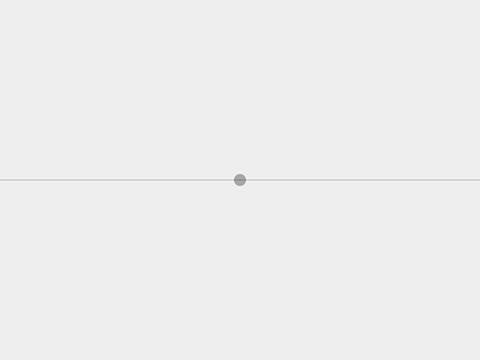// 初始化一個 800 x 600 大小的 PIXI.Application
var app = new PIXI.Application(800, 600, {backgroundColor : 0xEEEEEE});
document.body.appendChild(app.view);

// 本例子適合把 stage 放在畫面正中間
app.stage.x = app.renderer.width * 0.5;
app.stage.y = app.renderer.height * 0.5;

// 建立容器
var objContainer = new PIXI.Container();

// 畫球
var ball = new PIXI.Graphics();
ball.beginFill(0x000000, .3);
ball.drawCircle(0, 0, 10);
ball.endFill();

// 畫線
var objLine = new PIXI.Graphics();
objLine.lineStyle ( 1, 0x000000 , 0.3);
objLine.moveTo(-400, 0);
objLine.lineTo(400, 0);

Step2. 讓球動：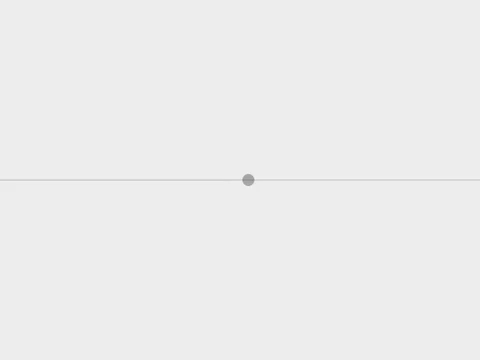var tk = 0;
tk ++;
if(tk == 360) { tk = 0; };
//讓球做簡諧運動
ball.x = Math.sin(tk  / (180 / Math.PI)) * (800 * 0.5);
});

800 是 PIXI.Application 的寬
800 * 0.5 是球會運動到左右邊界，也就是 -400 ~ 400 之間

Step3. 複製線段與球：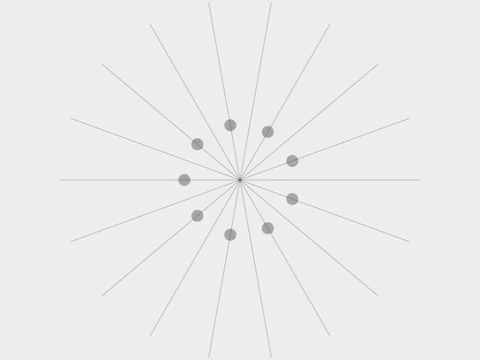var objCount = 9; // 會複製九段線與球
for(var i=1; i<objCount +1; i++){
this["objContainer"+i] = new PIXI.Container();
// … 畫出球與線段的部分與前面相同
this["objContainer"+i].rotation = (360 / objCount) * (i-1) * (Math.PI / 180);
}

90 * (Math.PI / 180);

Step4. 調整運動的參數var tk = 0;
tk ++;
if(tk == 360) { tk = 0; };
for(var i=1; i < objCount + 1; i++){
app.stage.children[i].children.x= 800 * Math.sin(( tk + (360 / objCount) * i )  / (180 / Math.PI)) * 0.5;
}
});

Math.sin(tk + 0 / (180 / Math.PI)) * (800 * 0.5);
Math.sin(tk + 120 / (180 / Math.PI)) * (800 * 0.5);
Math.sin(tk + 240 / (180 / Math.PI)) * (800 * 0.5);

Step5. 加入調整介面：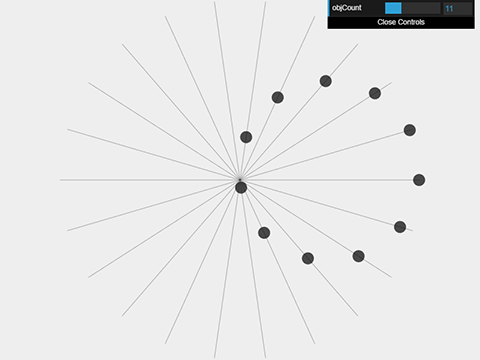Step5-1. 頁面上匯入 dat.gui.js：<script src='js/libs/dat.gui.min.js'></script>
Step5-2. 頁面裡的程式碼：

var gui = new dat.GUI();
var effectController = {
objCount: objCount
};

// 滑動的 controller，拖動範圍為 3~15，間隔為1
gui.add( effectController, "objCount", 3, 15, 1 ).onChange( countChange );

function countChange(){ // 拖動時會執行的程式
rebuildObjs();
};

function rebuildObjs(){
... //把頁面上的元件都清掉，並重新複製線段與線
}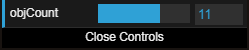gui.add( effectController, "objCount", 3, 15, 1 ).onChange( countChange );
controller 對應到 effectController 裡的 objCount 物件，最小值 3，最大值 15，每次拖動的間隔為 1；拖動結果為 3, 4, 5, 6… 15

http://eia.github.io/pixijs/170317/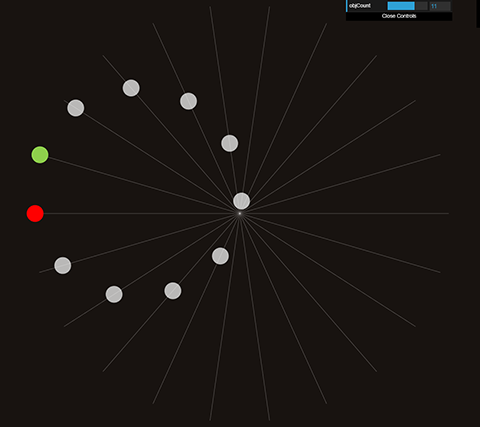resize：

app.stage.x = app.renderer.width * 0.5;
app.stage.y = app.renderer.height * 0.5;

• 手機旋轉，因為線段與球的邊界其實是頁面長寬裡比較短的邊，所以多給了一個變數並在 resize 時更新
• 手機版調整 app.stage 的 scale 為 2

1 則留言

0